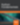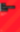# JavaScript Object-Oriented Programming Part 1

JavaScript

Share:

#### Free JavaScript Book!Write powerful, clean and maintainable JavaScript.

RRP \$11.95

## Keeping Things Tidy

Let’s say we want to keep all our properties and methods in the same place – in the `Circle()` constructor function. There are many ways to do this. Let’s first examine inner functions. An inner function is a function within a function (say that sentence quickly ten times!). Here’s what they let us do:

``````function Circle(radius){
function getArea(){
}
function getCircumference(){
var circumference = diameter*3.14;
return circumference;
}
this.getArea = getArea;
this.getCircumference = getCircumference;
}``````

It’s the same code, except that we’ve moved the functions. Now, inside our two functions, instead of `this.radius`, we could use just plain old `radius` because inner functions can access local variables within outer functions. Thus, it would be able to access the `radius` local variable passed as an argument to the `Circle() constructor`. Therefore, we could have just as easily used:

``````function Circle(radius){
function getArea(){
}
function getCircumference(){
var circumference = diameter*3.14;
return circumference;
}
this.getArea = getArea;
this.getCircumference = getCircumference;
}``````

Ok, now let’s change the `radius` of an object and get its area:

``````bigCircle.radius=50;

But wait! It returns 31400, rather than the expected 7850. What’s wrong? Well, `radius` refers to the value we passed to the `Circle()` constructor function, not the value of the object. So when we change the object’s `radius`, the methods `getArea()` and `geCircumference()`, keep on using the old radius. So, we really shouldn’t use just plain old `radius`. Instead, we need to use `this.radius`, as it refers to the current object’s radius, whether this property changes after the object is created or not.

Ok, so now we’ve created a self-contained object constructor – the function that defines an object. Let’s look at another way we can create functions inside our `Circle()` constructor:

``````function Circle(radius){
this.getArea = function(){
}
this.getCircumference = function(){
var circumference = diameter*3.14;
return circumference;
}
}

var bigCircle = new Circle(100);
var smallCircle = new Circle(2);

Here, we’ve encountered another way to define a function. We can use:

``````functionName = function([parameters]){
// function body
}``````

In this way, we can create parameters:

``````functionName = function(parameter1,parameter2,parameter3){
//function body
}``````

While functions aren’t created this way very often, when we’re creating objects, they can be useful shortcuts. These processes also help avoid conflicts with function names. For instance, another object can have a different function with the same name, for example `getArea()`, without causing a conflict. This is possible because these functions are encapsulated inside an object constructor.

Go to page: 1 | 2 | 3 | 4### Ryan Frishberg

Ryan has been involved with client-side scripting since 1999 when he started high school. He was part of the Flex SDK team and is now a software consultant.

#### New books out now!Learn valuable skills with a practical introduction to Python programming!Give yourself more options and write higher quality CSS with CSS Optimization Basics.